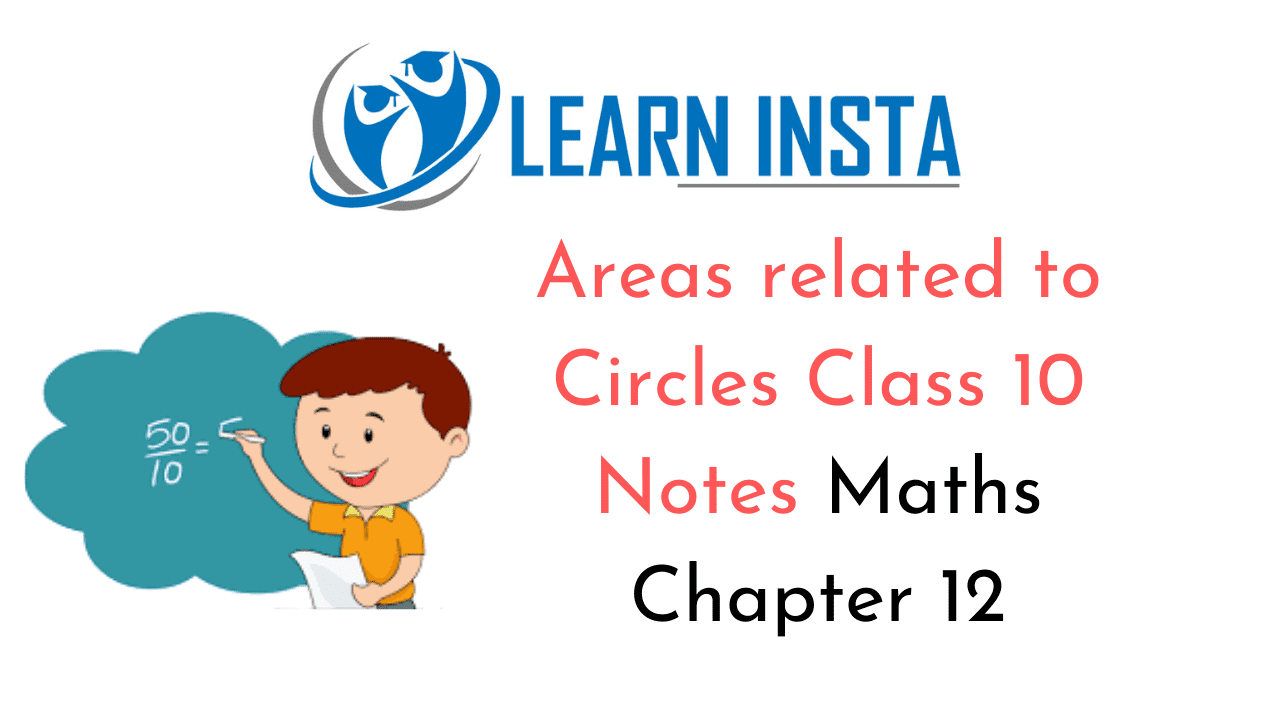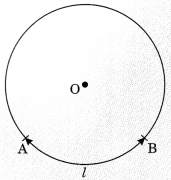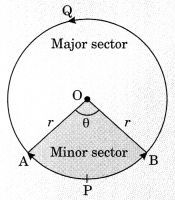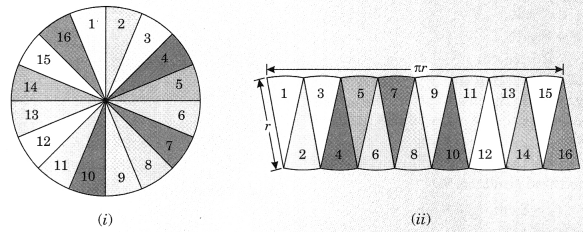On this page, you will find Areas related to Circles Class 10 Notes Maths Chapter 12 Pdf free download. CBSE NCERT Class 10 Maths Notes Chapter 12 Areas related to Circles will seemingly help them to revise the important concepts in less time.

## CBSE Class 10 Maths Chapter 12 Notes Areas related to Circles

### Areas related to Circles Class 10 Notes Understanding the Lesson

1. Circle: Circle is set of all points in a plane which are at the fixed distance from a fixed point i.e., centre. Centre: Mid-point of a circle is called centre of a circle.

2. Radius: The distance between the centre of a circle to the circumference of the circle.
It is denoted by r or R.

3. Chord: A line segment which joins the two points in the circumference of the circle.

4. Diameter: It is the longest chord which passes through the centre of a circle. It is denoted by d or D.
$$\text { Radius }=\frac{\text { Diameter }}{2}$$

5. Circumference of a Circle (or Perimeter)
A perimeter is a path that surrounds a two dimensional shape.
The perimeter of a circle is called its circumference. Circumference
$$\frac{\text { Circumference }}{\text { Diameter }}=\pi$$
Circumference = n x diameter = π x 2r = 2πr
or
The value of π is $$\frac{22}{7}$$ or 3.14 (approximately)

6. Arc: An arc is the part of the circumference of a circle.
An arc AB is denoted as $$\widehat{\mathrm{AB}}$$Length of arc AB is l $$\widehat{\mathrm{AB}}$$ or l.
Length of an arc of a sector of angle
$$\theta=\frac{2 \pi r \theta}{360^{\circ}}$$

7. Sector: The portion (or part) of circular region enclosed by two radii and the corresponding arc is called a sector of the circle.

8. Minor and Major sector of the circle: Shaded region OAPB is called sector or minor sector of a circle with centre O.
∠AOB is called angle of the sector.And OAQB is called major sector.
Angle of major sector = 360° – ∠AOB.

9. Area of CircleLet r be the radius of circle. If we cut the circle in sectors and arrange then we see this figure like a rectangle whose length is $$\frac{1}{2}$$ 2πr = πr and breadth is r.
Hence,
Area of circle = Area of rectangle
= l x b = $$\frac{1}{2}$$ 2πr = r
Area of circle = πr2

10. Segment: The portion or part of a circular region enclosed between a chord and the corresponding arc is called a segment of the circle.
The shaded region APB is the minor segment.
And the region AQB is the major segment.
Area of circle = πr2
Area of the sector of an angle $$\theta=\frac{\pi r^{2} \theta}{360^{\circ}}$$Area of major sector = Area of circle – Area of minor sector
Area of minor segment = Area of sector – Area of ΔOAB$$\frac{\pi r^{2} \theta}{360^{\circ}}$$– area of ΔOAB
Area of major segment = Area of circle – Area of minor segment

11. Area of Combinations of Plane Figures
In our daily life we have observed various plane figures which are combinations of two or more figures and i also in the form of various interesting designs like flower beds, curtains, drain covers, window designs, ] designs on table covers.  To calculate areas of such figures, we field the area of shapes used and then and/subtract as per need.